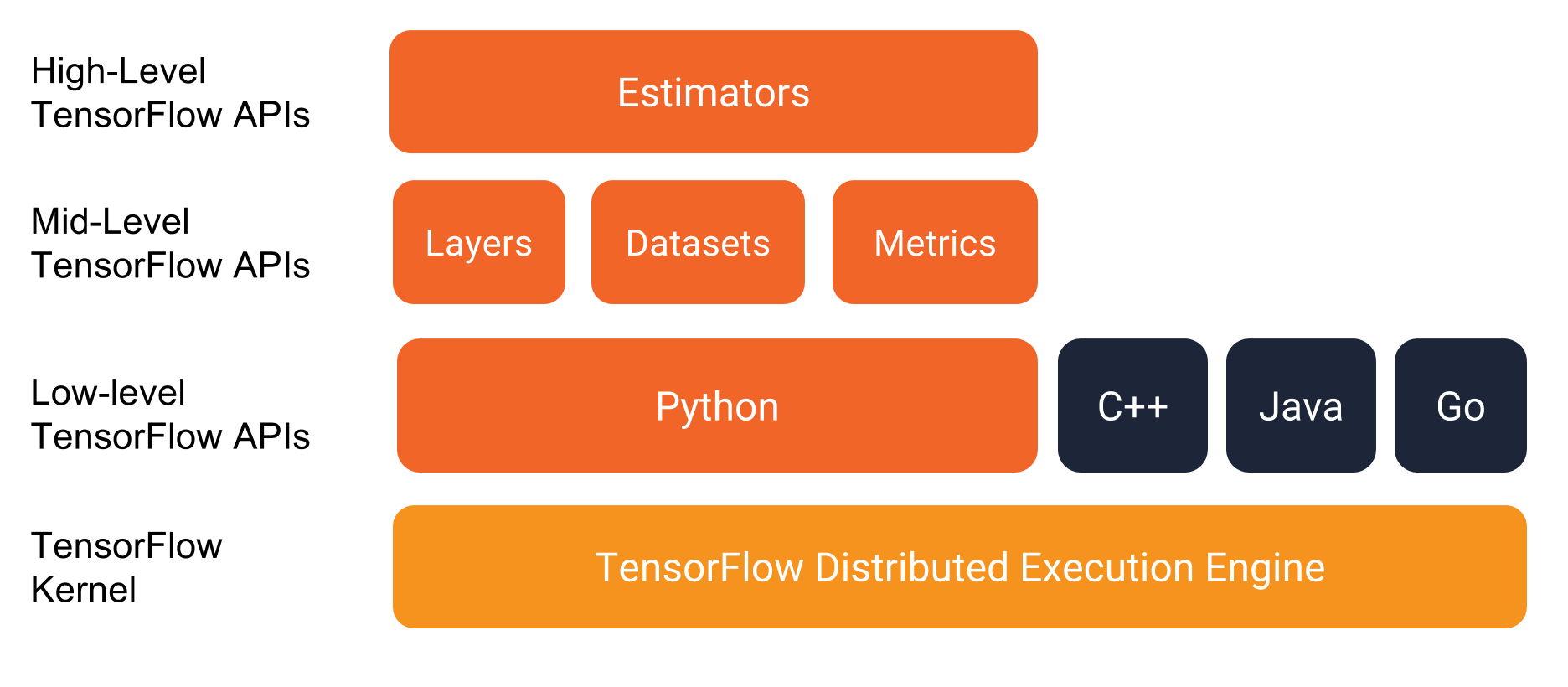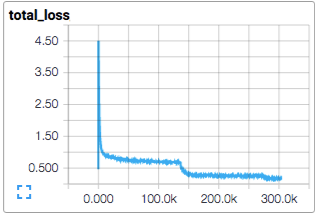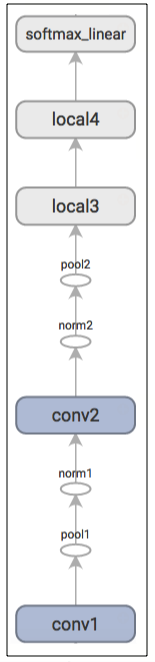Deep Learning Frameworks

Building Convolutional Neural Networks on TensorFlow: Three Examples

Convolutional Neural Networks (CNN) are the foundation of computer vision and image classification. TensorFlow lets you build CNN architectures with tremendous flexibility, but can be a bit challenging at first.

In this article we refresh basic concepts you’ll need to build CNNs on TensorFlow, and provide an overview of three neural network examples:

Scaling Up CNN on TensorFlow with MissingLink

If you’re working on a CNN project, you probably have a large dataset and need to run your experiments on several machines.

This can become challenging, and you might find yourself spending serious time on setting up machines, copying data and troubleshooting, instead of building a winning experiment.

MissingLink is a deep learning platform that lets you effortlessly scale TensorFlow CNN models across many machines, either on-premise or in the cloud. It also helps you manage large data sets, manage multiple experiments, and view metrics and hyperparameters across your team on one convenient dashboard.

Concepts Required to Understand CNN on TensorFlow

What is a tensor?

A tensor is a way to represent deep learning data. It is a multidimensional array, used to store data for multiple features of a dataset, where each feature represents an additional dimension. For example, a 3-dimensional tensor is a “cube” storing values along three axes.What is a computational graph?

The TensorFlow computational graph represents the flow of operations that occur during training of a deep learning model. For CNN models, the computational graph can be quite complex. Below is an example of a simple graph. You can visualize your model’s computational graph using TensorBoard – learn more about TensorFlow visualization .What is a constant?

Used in TensorFlow to store constant values that don’t change. Used for nodes that are required to stay the same throughout model training. A constant does not take inputs.

What is a placeholder?

Used to feed input when running a model. A placeholder can take parameters, and so it can be modified in runtime, while you are running the computational graph.

What is a variable?

Used to modify the computational graph, adding parameters or nodes to the graph which are trainable.

TensorFlow API levels

TensorFlow lets you work directly with Tensors to build a neural network from the ground up. However, instead of using these low-level APIs which can be quite complex, TensorFlow recommends working with the higher-level Estimators API. This allows you to define an object, at a higher level of abstraction, which creates and trains deep learning structures.Image Source: TensorFlow

Basic TensorFlow CNN Example: Using MNIST Dataset with Estimators

A great way to get started with CNN on TensorFlow is to work with examples based on standard datasets. These datasets are built into TensorFlow and will give you predictable results, helping you learn to run and tune a model.

The TensorFlow MNIST example builds an Estimator that creates a Convolutional Neural Network, which can classify handwritten digits in the MNIST dataset. Below are the general steps;

Architecture:

1. Convolutional layer with 32 5×5 filters
2. Pooling layer with 2×2 filter
3. Convolutional layer with 64 5×5 filters
4. Pooling layer with 2×2 filter
5. Dense layer with 1024 neurons
6. Dense layer with 10 neurons, to predict the digit for the current image

Process:

1. Build the input layer using the reshape() function.
2. Build the convolutional/pooling layers using the layers.conv2d()  and layers.max_pooling2d() functions.
3. Build the dense layers using the layers.dense() function.
4. Generate predictions by running the softmax() function.
5. Calculate loss by running the losses.sparse_softmax_cross_entropy() function.
6. Configure the training operation using the optimizer.minimize() function.
7. Add an evaluation metric using tf.metrics.accuracy()
9. Define an Estimator for the model (the example provides a ready-made estimator for MNIST data).
10. Train the model by running the train() function on the Estimator object.
11. Evaluate the model on all MNIST images using the evaluate() function.

Example code and tutorial: https://www.tensorflow.org/tutorials/estimators/cnn

Intermediate TensorFlow CNN Example: Fashion-MNIST Dataset with Estimators

This is a slightly more advanced example using 28×28 grayscale images of 65,000 fashion products in 10 categories. The dataset was presented in an article by Xiao, Rasul and Vollgraf, and is not built into TensorFlow, so you’ll need to import it and perform some pre-processing.

Architecture: The model uses three convolutional layers:

1. Convolutional layer with 32-3 and 3 filters
2. Max pooling layer with 2×2 filter
3. Convolutional layer with 64-4 and 3 filters
4. Max pooling layer with 2×2 filter
5. Convolutional layer with 128-3 and 3 filters
6. Max pooling layer with 2×2 filter
7. Flattening
8. Dense layer with 128 neurons
9. Output layer with 10 neurons corresponding to the 10 fashion categoriesProcess:

1. Load data – requires one-hot encoding because the dataset is not built into TensorFlow.
2. Reshape images to 28x28x1.
3. Define network parameters and placeholders.
4. Build the network architecture using conv_net() function, conv2d() and maxpool2d().
5. Add loss and optimizer nodes.
7. Train and test the model.
8. Plot accuracy and loss between training and validation data.Source: TensorFlow

Example code and tutorial: https://www.datacamp.com/community/tutorials/cnn-tensorflow-python

Advanced TensorFlow CNN Example: CIFAR10 without Estimators

This example shows how to build a CNN on TensorFlow without an Estimator, using lower level APIs that give you much more control over network structure and parameters.

In this example, you classify an RGB 32×32 pixel image across 10 categories: airplane, automobile, bird, cat, deer, dog, frog, horse, ship, and truck. The example includes a multi-GPU version which will show you how to scale up your model.

Architecture: Alternating convolutions and nonlinearities, followed by fully connected layer, ending with a softmax classifier.

Process:

1. Crop images to 24×24 pixels and random distortions are applied to increase the dataset size.
2. Use the inference() function to compute predictions. The computation graph is as follows:Source: TensorFlow
3. Train the model using standard gradient descent (see our in-depth guide on Backpropagation ), to minimize the loss of the softmax regression function (see our guide on activation functions ).
4. Launch the model using the training script, which reports total loss every 10 steps and the processing speed for the last batch of data.
5. Evaluate the model using the evaluation script. It tests the model on all 10,000 images in the evaluation set of CIFAR-10, and displays accuracy.
6. Train the same model on multiple GPUs by running the separate GPU training script. This creates several replicas of the model and runs each of them on a different GPU, on a subset of the training data.

Example code and tutorial:

https://www.tensorflow.org/tutorials/estimators/cnn

Running CNN on TensorFlow in the Real World

In this article we explained how to run Convolutional Neural Networks on TensorFlow. When you start working on CNN projects, processing and generating predictions for real images, audio and video, you’ll run into some practical challenges:Tracking experiment progress, source code, and hyperparameters across multiple CNN experiments. CNNs can have many different architectures and parameter tweaks, and testing each of these will require running and tracking large numbers of experiments.Running experiments across multiple machines—a CNN is computationally intensive, and projects will likely require multiple machines or GPUs. Provisioning these machines, distributing experiments on them and monitoring experiments as they run can become a burden.Manage training data—CNN projects usually involve images or video, and training sets can get huge, up to Gigabytes or Petabytes of data. Copying this data to each training machine and replacing it for different experiments can be time consuming and error prone.

MissingLink is a deep learning platform that can help you automate these operational aspects of CNN on TensorFlow, so you can concentrate on building winning experiments. Learn more.